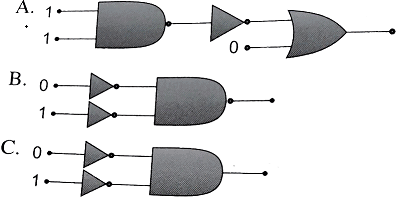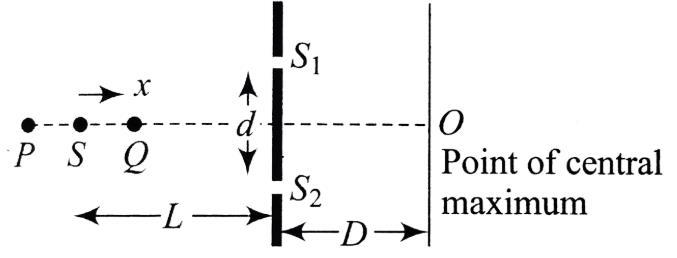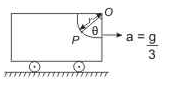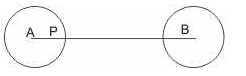Courses

# JEE(MAIN) Physics Mock Test - 7

## 30 Questions MCQ Test JEE Main Mock Test Series 2020 & Previous Year Papers | JEE(MAIN) Physics Mock Test - 7

Description
This mock test of JEE(MAIN) Physics Mock Test - 7 for JEE helps you for every JEE entrance exam. This contains 30 Multiple Choice Questions for JEE JEE(MAIN) Physics Mock Test - 7 (mcq) to study with solutions a complete question bank. The solved questions answers in this JEE(MAIN) Physics Mock Test - 7 quiz give you a good mix of easy questions and tough questions. JEE students definitely take this JEE(MAIN) Physics Mock Test - 7 exercise for a better result in the exam. You can find other JEE(MAIN) Physics Mock Test - 7 extra questions, long questions & short questions for JEE on EduRev as well by searching above.
QUESTION: 1

Solution:
QUESTION: 2

### A hotwire ammeter reads 10 A in a a.c. circuit. The peak value of the current is

Solution:

Hot wire ammeter always gives rms value of current, i.e.,
Irms = 10 amp. as Irms = I0/√2

hence I= √2 Irms = √2 x 10 amp = 10√2 A

QUESTION: 3

### The average acceleration vector for a particle having a uniform circular motion is

Solution: In uniform circular motion (UCM), the direction of the acceleration vector points toward the centre of the circle. However, it constantly changes with time. The average of these vectors over one cycle is a null vector.
QUESTION: 4
The X-rays beams coming from an X-ray tube will be
Solution:
QUESTION: 5

A dipole of dipole moment p̅ is placed in uniform electric field E̅, then torque acting on it is given by

Solution:
QUESTION: 6
When two surfaces are coated with a lubricant, then they
Solution:
QUESTION: 7
Two pieces of a glass plate, placed one upon the other with a little water in between them, can not be separated easily, because of
Solution:
QUESTION: 8

The gyromagnetic ratio of an electron of charge e and mass m is equal to

Solution:
QUESTION: 9
If the momentum of a body increases by 0.01% its kinetic energy will increase by
Solution:
QUESTION: 10

An electric dipole is placed along the X-axis at the origin O. A point P is at a distance of 20 cm from this origin such that OP makes an angle π/3 with the X axis. If electric field at P makes an angle θ with X-axis, the value of θ is

Solution:
QUESTION: 11

In the following combinations of logic gates, the outputs of A, B and C are respectivleySolution:
QUESTION: 12
Find the maximum velocity for overturn for a car moved on a circular track of radius 100 m. The coefficient of friction between the road and tyre is 0.2
Solution:
QUESTION: 13

An alpha nucleus of energy 1/2 mv2  bombards a heavy nuclear target of charge Ze. Then the distance of closest approach for the alpha nucleus will be proportional to

Solution:
QUESTION: 14
In the following question, a Statement of Assertion (A) is given followed by a corresponding Reason (R) just below it. Read the Statements carefully and mark the correct answer-
Assertion(A): We cannot think of a magnetic configuration with three poles.
Reason(R): A bar magnet does exert a torque on itself due to its own field.
Solution:
QUESTION: 15
In the following question, a Statement of Assertion (A) is given followed by a corresponding Reason (R) just below it. Read the Statements carefully and mark the correct answer-
Assertion(A): Gravitational field intensity at the centre of ring is zero.
Reason(R): Net force at the centre of ring is zero.
Solution:
QUESTION: 16

A disc is of mass M and radius r. The moment of inertia of it about an axis tangential to its edge and in plane of the disc or parallel to its diameter is

Solution:
QUESTION: 17

Helium gas at 27oC has a volume of 8 L. It is suddenly compressed to a volume of 1 L. The final temperature of the gas will be(γ = 5/3 )

Solution:

T1 V1 γ-1 = T2 V2 γ-1
or 300 (8)5/3 - 1 = T2 (1)5/3 - 1
T2 = 300 {(2)3}2/3 = 1200 K
or T2 = 927oC

QUESTION: 18
A tuning fork produces waves in a medium. If the temperature of the medium changes, then which of the following will change
Solution:
QUESTION: 19
Solution:
QUESTION: 20

The radionuclide 56Mn is being produced in a cyclotron at a constant rate P by bombarding a manganese target with deutrons. 56Mn has a half life of 2.5 hours and the target contains large number of only the stable manganese isotope 55Mn. The reaction that produces 56Mn is:

55Mn + d 56Mn+P

After being bombarded for a long time, the activity of 56Mn becomes constant equal to 13.86×1010s−1. (Use ln2 = 0.693; Avogadro number = 6×1023; atomic weight of 56Mn = 56gm/mole)

Q. After a long time bombardment, number of 56Mn nuclei present in the target depends upon
(a) The number of 56Mn nuclei present at the start of the process
(b) Half life of the 56Mn
(c) The constant rate of production P

Solution:
QUESTION: 21

The radionuclide 56Mn is being produced in a cyclotron at a constant rate P by bombarding a manganese target with deutrons. 56Mn has a half life of 2.5 hours and the target contains large number of only the stable manganese isotope 55Mn. The reaction that produces 56Mn is:

55Mn + d 56Mn+P

After being bombarded for a long time, the activity of 56Mn becomes constant equal to 13.86×1010s−1. (Use ln2 = 0.693; Avogadro number = 6×1023; atomic weight of 56Mn = 56gm/mole)

Q. At what constant rate P , 56Mn nuclei are being produced in the cyclotron during the bombardment?

Solution:
QUESTION: 22

In an Young's double slit experiment setup, as shown in the figure, the light source executes simple harmonic motion between P and Q according to the equation x = Asinωtx, s being the mean position. Assume d→O and A≪L. ω is small enough to neglect Doppler effect. If the source were stationary at s, intensity at O would be I0.Q. When the source comes towards the point Q,

Solution:
QUESTION: 23

In an Young's double slit experiment setup, as shown in the figure, the light source executes simple harmonic motion between P and Q according to the equation x = Asinωtx, s being the mean position. Assume d→O and A≪L. ω is small enough to neglect Doppler effect. If the source were stationary at s, intensity at O would be I0.

Q. The fringe width β can be expressed as

Solution:
QUESTION: 24

In an Young's double slit experiment setup, as shown in the figure, the light source executes simple harmonic motion between P and Q according to the equation x = Asinωtx, s being the mean position. Assume d→O and A≪L. ω is small enough to neglect Doppler effect. If the source were stationary at s, intensity at O would be I0.

Q. The fractional change in intensity of the central maximum as function of time is

Solution:
QUESTION: 25

An electron - volt is the unit of

Solution:
QUESTION: 26

Three different capacitors are connected in series. Then:

Solution:
QUESTION: 27

A body at point P is free to slide with negligible friction on the circular guide mounted in the vertical frame as shown in figure. If the frame starts from rest with a constant acceleration. The velocity of body as a function of θ isSolution:
QUESTION: 28

If current in a source of e.m.f. is in the direction of e.m.f., the energy of the source

Solution:
QUESTION: 29

A charged particle moving in a magnetic field experiences a resultant force

Solution:
QUESTION: 30

Two identical thin non-conducting spherical shells of radius R carry equal negative charges of magnitude Q sprayed uniformly on their surfaces. The distance AB between their centres is 4R. The electric field at a point P(fig.) at distance R/2 from the centre A of the first sphere isSolution: